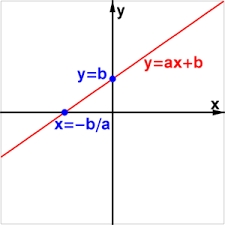# Suppose

Suppose you know that the length of a line segment is 15, x2=6, y2=14 and x1= -3. Find the possible value of y1. Is there more than one possible answer? Why or why not?

Result

y11 =  26
y12 =  2

#### Solution:

$x_{2}=6 \ \\ y_{2}=14 \ \\ x_{1}=-3 \ \\ \ \\ d=15 \ \\ (x_{1}-x_{2})^2 +(y_{1}-y_{2})^2=d^2 \ \\ (-3-6)^2 +(y_{1}-14)^2=15^2 \ \\ (-3-6)^2 +(y_{1}-14)^2=15^2 \ \\ \ \\ \ \\ q^2 -28q +52=0 \ \\ \ \\ a=1; b=-28; c=52 \ \\ D=b^2 - 4ac=28^2 - 4\cdot 1 \cdot 52=576 \ \\ D>0 \ \\ \ \\ q_{1,2}=\dfrac{ -b \pm \sqrt{ D } }{ 2a }=\dfrac{ 28 \pm \sqrt{ 576 } }{ 2 } \ \\ q_{1,2}=\dfrac{ 28 \pm 24 }{ 2 } \ \\ q_{1,2}=14 \pm 12 \ \\ q_{1}=26 \ \\ q_{2}=2 \ \\ \ \\ \text{ Factored form of the equation: } \ \\ (q -26) (q -2)=0 \ \\ \ \\ y_{11}=q_{1}=26$

Checkout calculation with our calculator of quadratic equations.

$y_{12}=q_{2}=2$Our examples were largely sent or created by pupils and students themselves. Therefore, we would be pleased if you could send us any errors you found, spelling mistakes, or rephasing the example. Thank you!

Leave us a comment of this math problem and its solution (i.e. if it is still somewhat unclear...):

Showing 1 comment:Matematik
we make circle k with centre S(x2,y2) and radius r = 15 . Then we make vertical line x= -3 . It make two intersections with circle k thus solutions are two: y11,y12.Tips to related online calculators
For Basic calculations in analytic geometry is helpful line slope calculator. From coordinates of two points in the plane it calculate slope, normal and parametric line equation(s), slope, directional angle, direction vector, the length of segment, intersections the coordinate axes etc.
Looking for help with calculating roots of a quadratic equation?
Do you have a linear equation or system of equations and looking for its solution? Or do you have quadratic equation?
Do you want to convert length units?
Pythagorean theorem is the base for the right triangle calculator.

## Next similar math problems:Show that the quadrilateral with vertices P1(0,1), P2(4,2) P3(3,6) P4(-5,4) has two right triangles.
2. Trapezoid MO-5-Z8ABCD is a trapezoid that lime segment CE divided into a triangle and parallelogram as shown. Point F is the midpoint of CE, DF line passes through the center of the segment BE and the area of the triangle CDE is 3 cm2. Determine the area of the trapezoid
3. Ratio of sidesCalculate the area of a circle that has the same circumference as the circumference of the rectangle inscribed with a circle with a radius of r 9 cm so that its sides are in ratio 2 to 7.
4. RectangleIn rectangle with sides, 6 and 3 mark the diagonal. What is the probability that a randomly selected point within the rectangle is closer to the diagonal than to any side of the rectangle?
5. Eq triangle minus arcsIn an equilateral triangle with a 2cm side, the arcs of three circles are drawn from the centers at the vertices and radii 1cm. Calculate the content of the shaded part - a formation that makes up the difference between the triangle area and circular cuts
6. Squares above sidesTwo squares are constructed on two sides of the ABC triangle. The square area above the BC side is 25 cm2. The height vc to the side AB is 3 cm long. The heel P of height vc divides the AB side in a 2: 1 ratio. The AC side is longer than the BC side. Calc
7. The trapeziumThe trapezium is formed by cutting the top of the right-angled isosceles triangle. The base of the trapezium is 10 cm and the top is 5 cm. Find the area of trapezium.
8. Annular areaThe square with side a = 1 is inscribed and circumscribed by circles. Find the annular area.
9. 30-gonAt a regular 30-gon the radius of the inscribed circle is 15cm. Find the "a" side size, circle radius "R", circumference, and content area.
10. The sides 2The sides of a trapezoid are in the ratio 2:5:8:5. The trapezoid’s area is 245. Find the height and the perimeter of the trapezoid.
11. Area of a rectangleCalculate the area of a rectangle with a diagonal of u = 12.5cm and a width of b = 3.5cm. Use the Pythagorean theorem.
12. Quarter circleWhat is the radius of a circle inscribed in the quarter circle with a radius of 100 cm?
13. Circular ringSquare with area 16 centimeters square are inscribed circle k1 and described circle k2. Calculate the area of circular ring, which circles k1, k2 form.
14. Trapezoid MOThe rectangular trapezoid ABCD with right angle at point B, |AC| = 12, |CD| = 8, diagonals are perpendicular to each other. Calculate the perimeter and area of ​​the trapezoid.
15. Hexagonal pyramidCalculate the surface area of a regular hexagonal pyramid with a base inscribed in a circle with a radius of 8 cm and a height of 20 cm.
16. Circular railwayThe railway is to interconnect in a circular arc the points A, B, and C, whose distances are | AB | = 30 km, AC = 95 km, BC | = 70 km. How long will the track from A to C?
17. Company logoThe company logo consists of a blue circle with a radius of 4 cm, which is an inscribed white square. What is the area of the blue part of the logo?